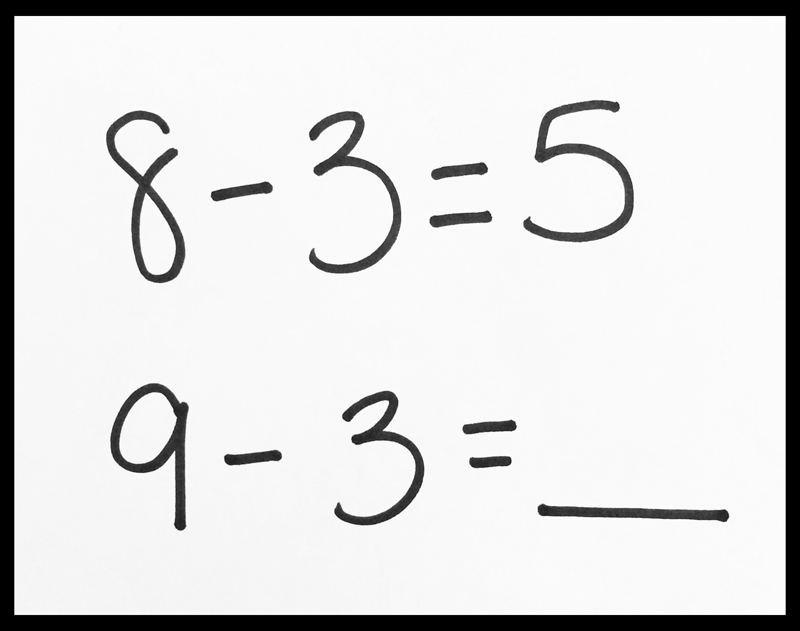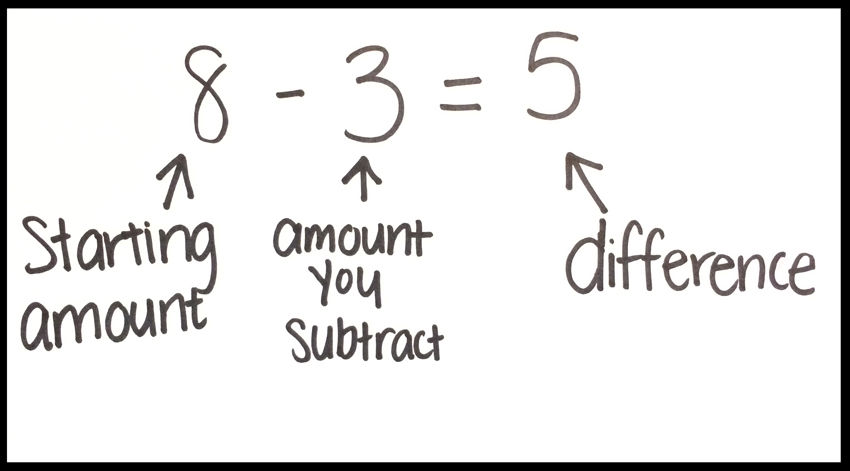Select Page

Using language to effectively communicate one’s mathematical thinking is an important skill—one that is a focus of Math Practice 6: Attend to Precision. Many of us know firsthand that clearly articulating mathematical ideas is challenging work, and that when students use ambiguous, imprecise terms in their explanations, their language can actually get in the way of understanding. Developing precise language is key if we want to students to engage in rich, collaborative discussions in which they can build on each other’s mathematical ideas, articulate arguments and develop conjectures. But what does it look like to do this work in the elementary grades?

Consider the following excerpt, taken from a second-grade classroom in which the teacher is working with the class to identify and articulate how the answer to a subtraction problem changes when the minuend (otherwise known as the first number) increases by 1. The teacher chooses to present pairs of problems with intentionally small numbers so that students can focus their full attention on exploring the properties of subtraction.

After discussing similar problems with the class, the teacher decides to introduce specific terms to help students describe the patterns that they are noticing across problems. Students have just completed a turn-and-talk about these problems and all students have agreed that the answer to the second problem is 6:Teacher: As we talk about these, I’m going to give you some new words to use. We’re going to call the first number the amount that you start with. We’re going to call the second number the amount that you subtract, and we’re going to call this the difference.Teacher: We’re going to try to use these words so that we are all clear about which parts of the problems we are describing as we share our thinking.

Let’s pause to consider the teacher’s moves. Notice that the teacher has chosen not to use the formal terms for the quantities in a subtraction problem (i.e. minuend, subtrahend), which tend to be quite confusing for students (and adults) and can actually get in the way of understanding the mathematics. Her purpose is to introduce phrases to promote the use of shared language among the students, and to use phrases that clearly describe the aspects of the problem students are considering.

With shared language introduced, the conversation continues…

Teacher: As you look at these problems, what do you notice about the starting amounts, the amount you subtract, and the difference?

Jessa: Um…well, the number you start with is increasing by 1 even though you are subtracting, it is going up by 1, it just matters what number you start with.

Caroline: Um, can I come up and show?

Teacher: Well, can you use the names of the numbers and use words like the amount you start with, the amount you subtract, and the difference?

Caroline: Um…

Caroline: Well, the amount that you start with is the same, and the thing you are taking away is the same, but what it is going to equal is not going to be the same, it is going to change to be 1 because it’s going to be 6…because…well can I come and show?

Teacher: What is staying the same in both problems?

Justin: The 3 is staying the same.

Teacher: So, the amount that we are subtracting is staying the same. Is that right?

Caroline: And then the amount that you start with increased by 1.

Teacher: And we agreed that the answer is 6. So, the difference also increased by 1?

Caroline: Yeah.

Like all elements of mathematics teaching and learning, using new mathematical vocabulary is hard work. It’s not easy for Caroline to integrate the new terms into her statement about what is changing and what is staying the same in both problems. Notice that Caroline claims that the starting amounts and the amount being subtracted are the same. In order to clarify this, the teacher asks students to state what quantity remains the same in both problems.

In using the phrases the “starting amount,” “amount you subtract,” and “difference,” the teacher supports students in considering how the quantities in subtraction situations relate to each other and promotes the use of shared language among the students. The teacher is purposeful, and her use of language reflects the learning goals she has for students.

This classroom excerpt illustrates that development of precise mathematical language begins in context, and like all language development, evolves gradually over time with repeated opportunities to experiment with language, explain thinking and listen to the explanations of others.

Tag(s): addition/subtraction | class discussion | classroom culture | grade 2 | mathematical vocabulary | teacher moves | The Math Practices |